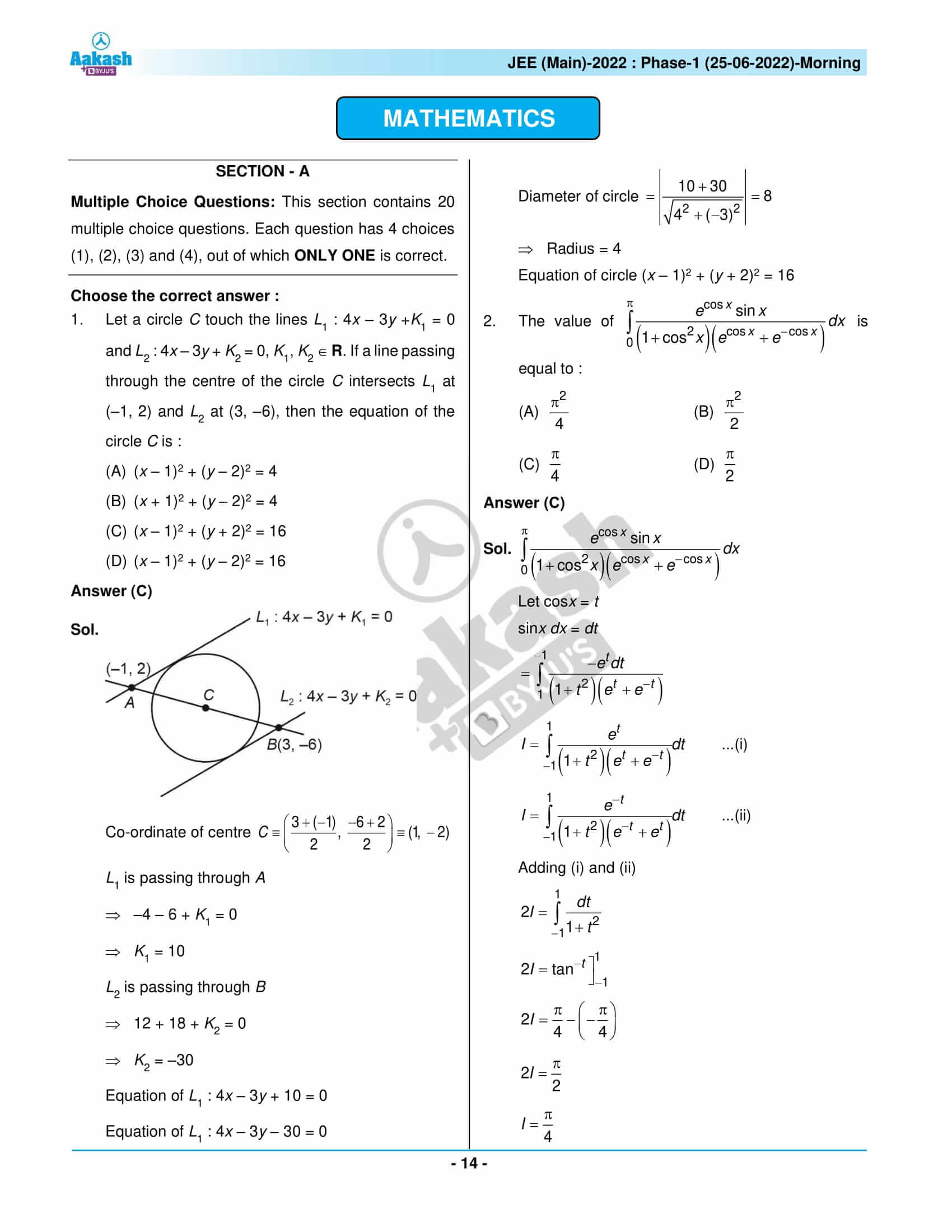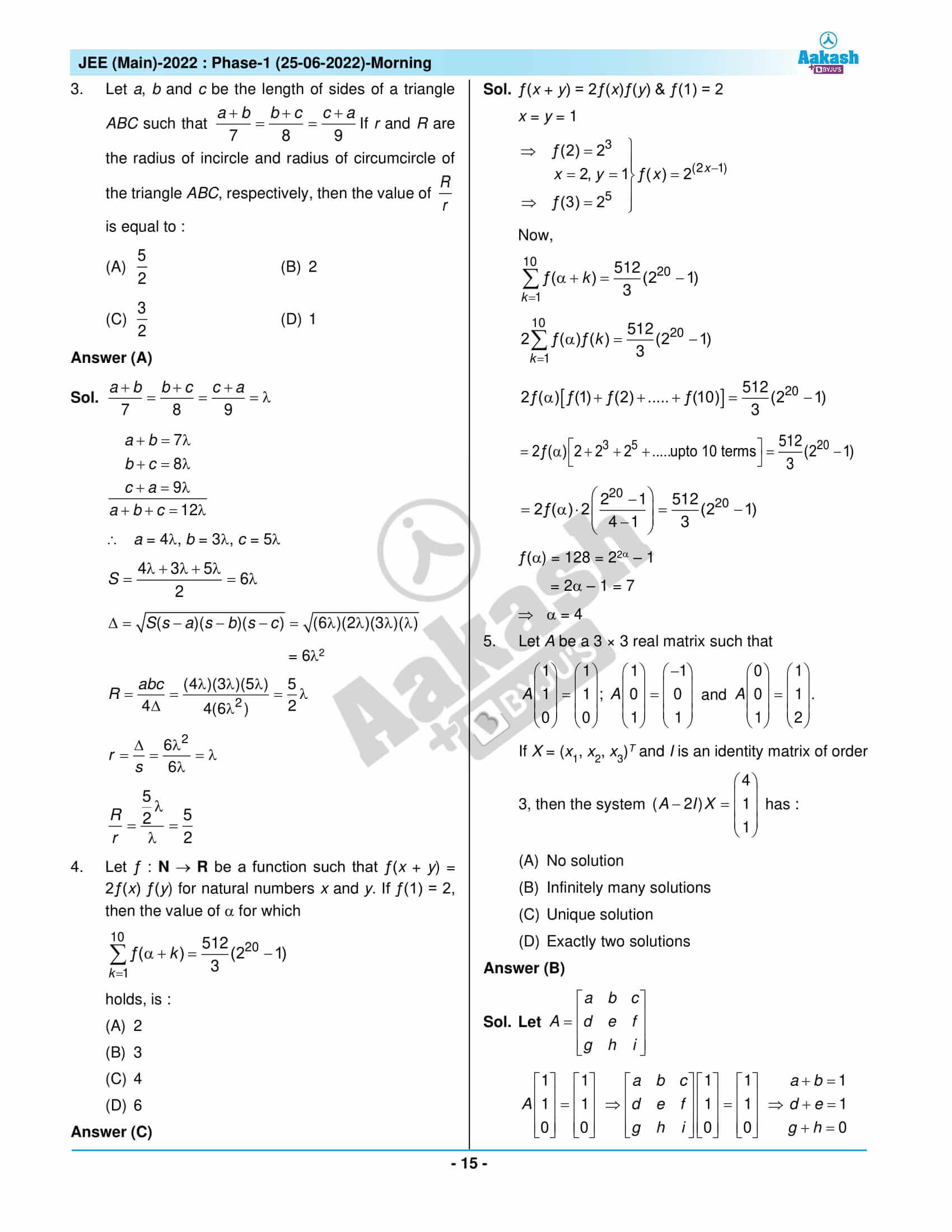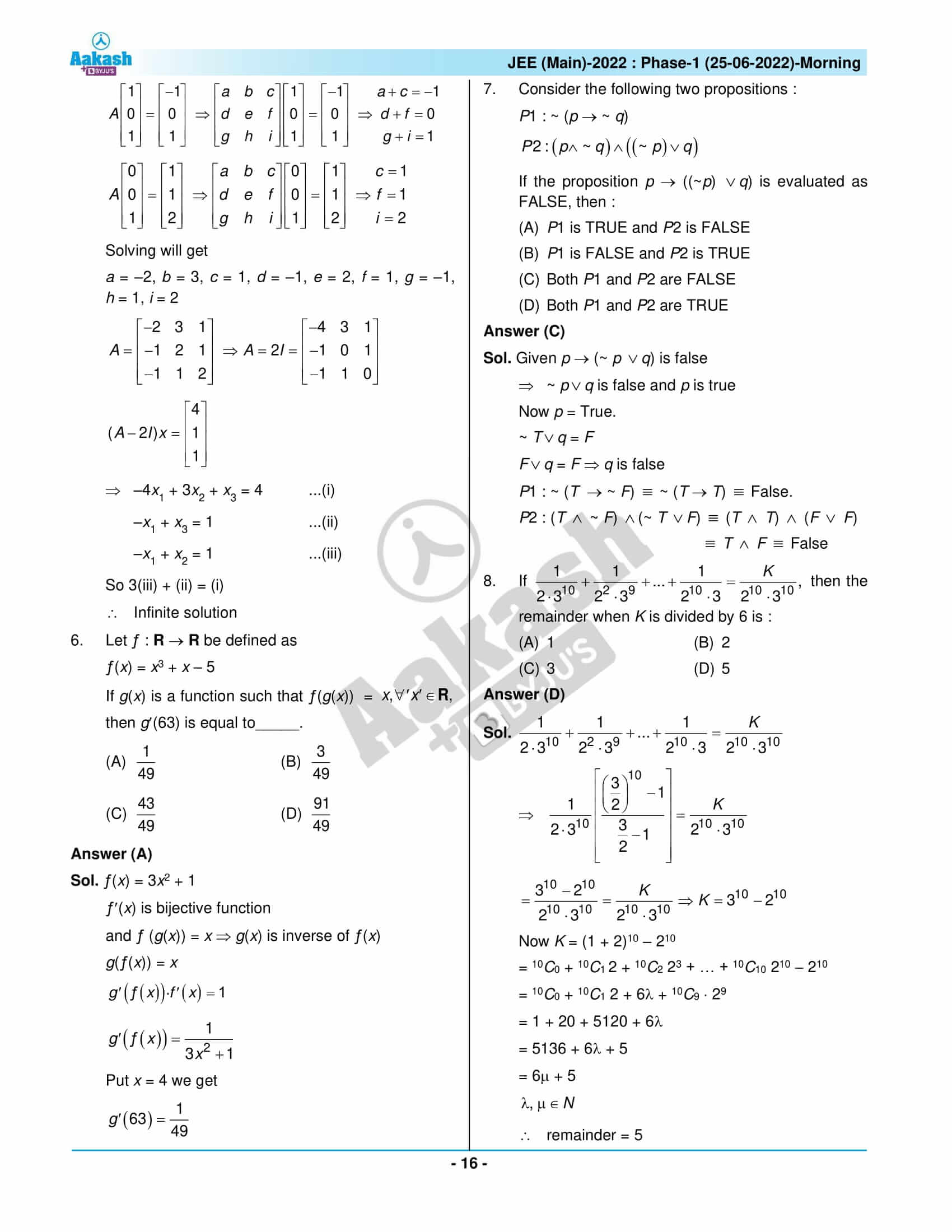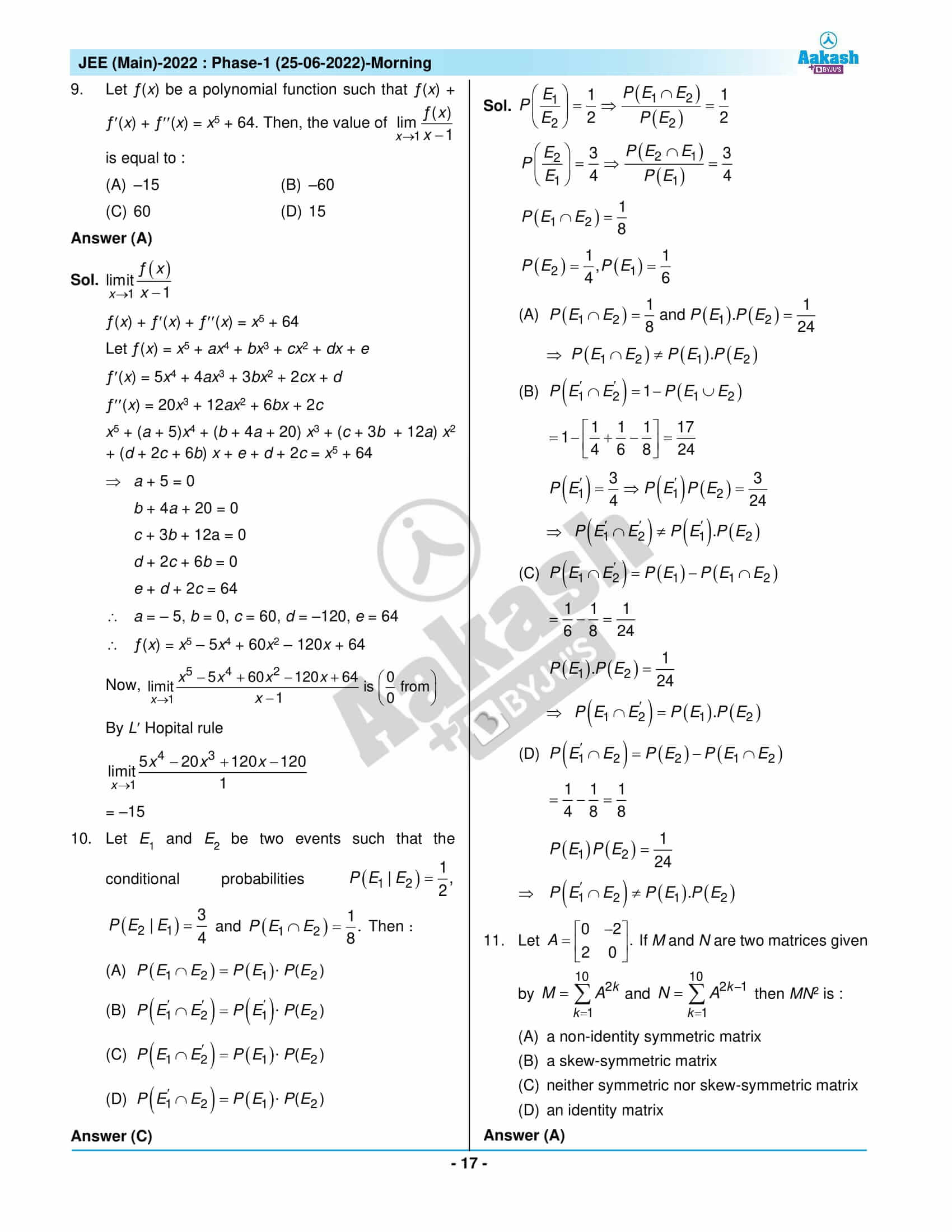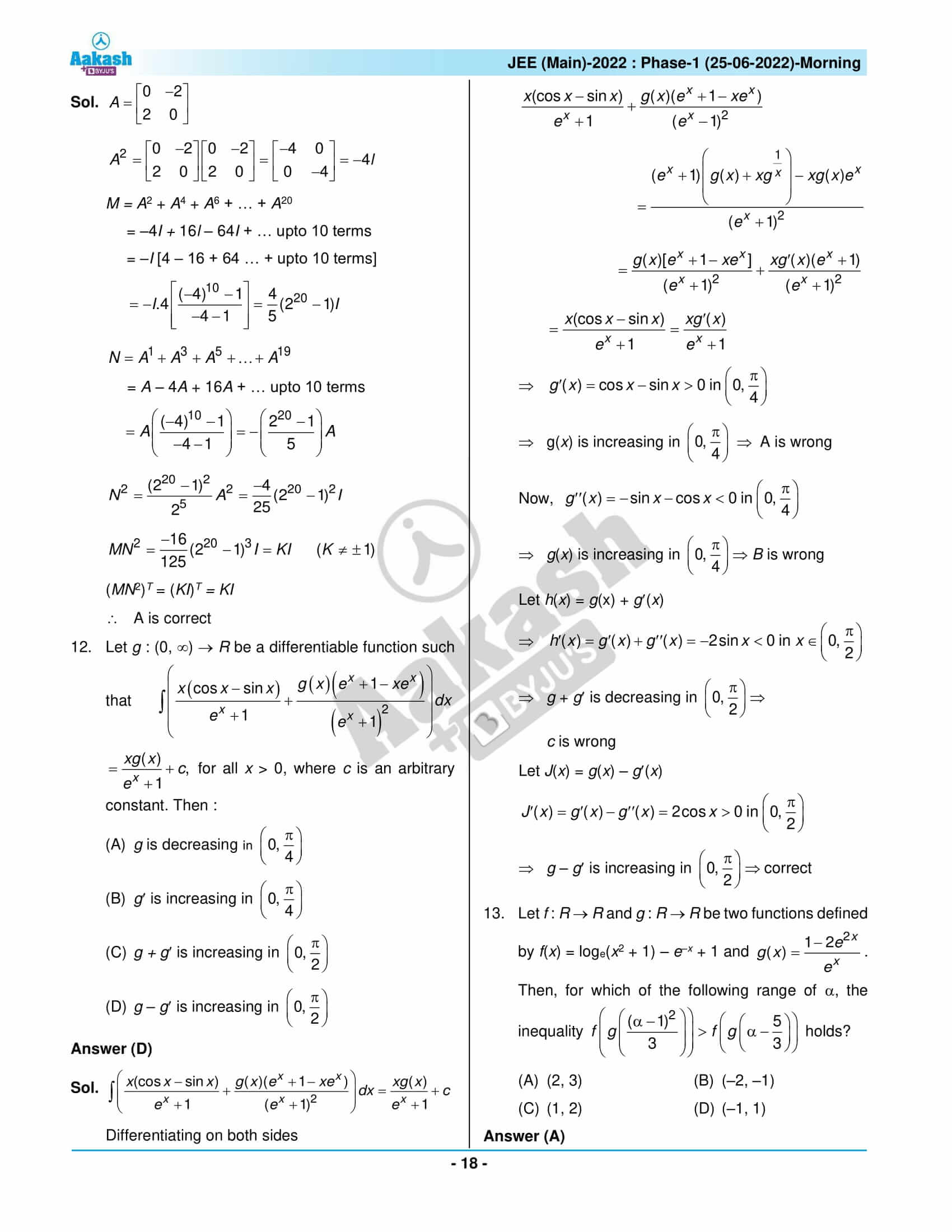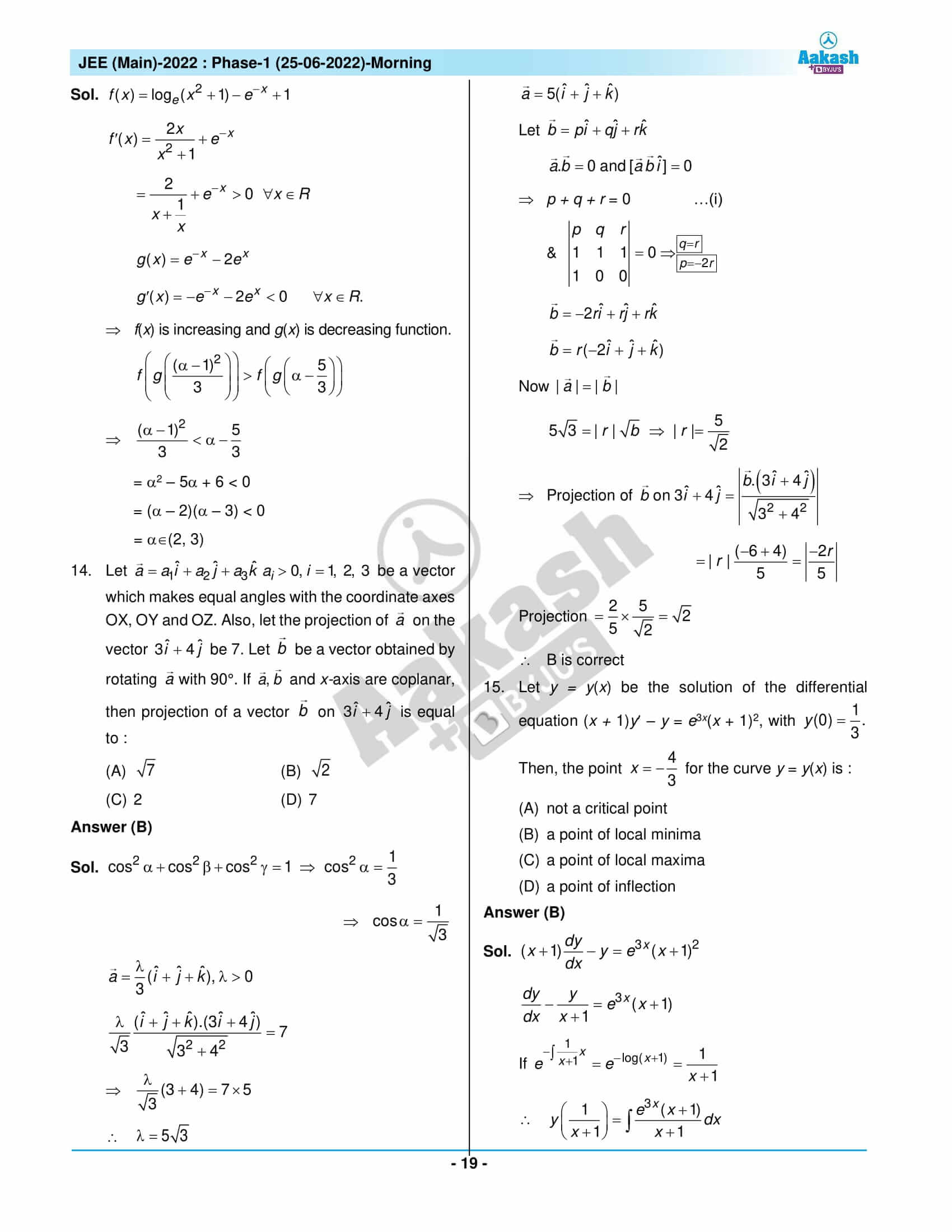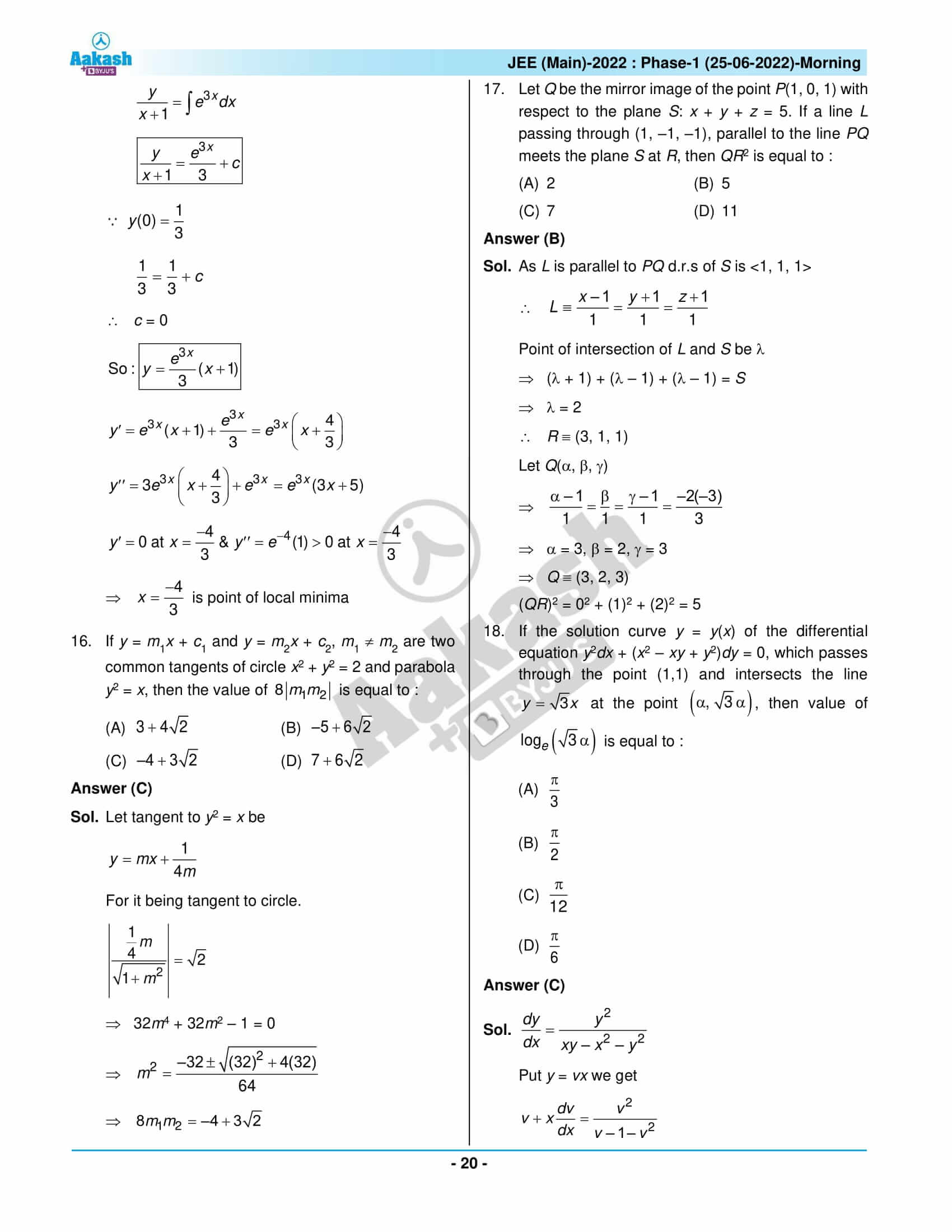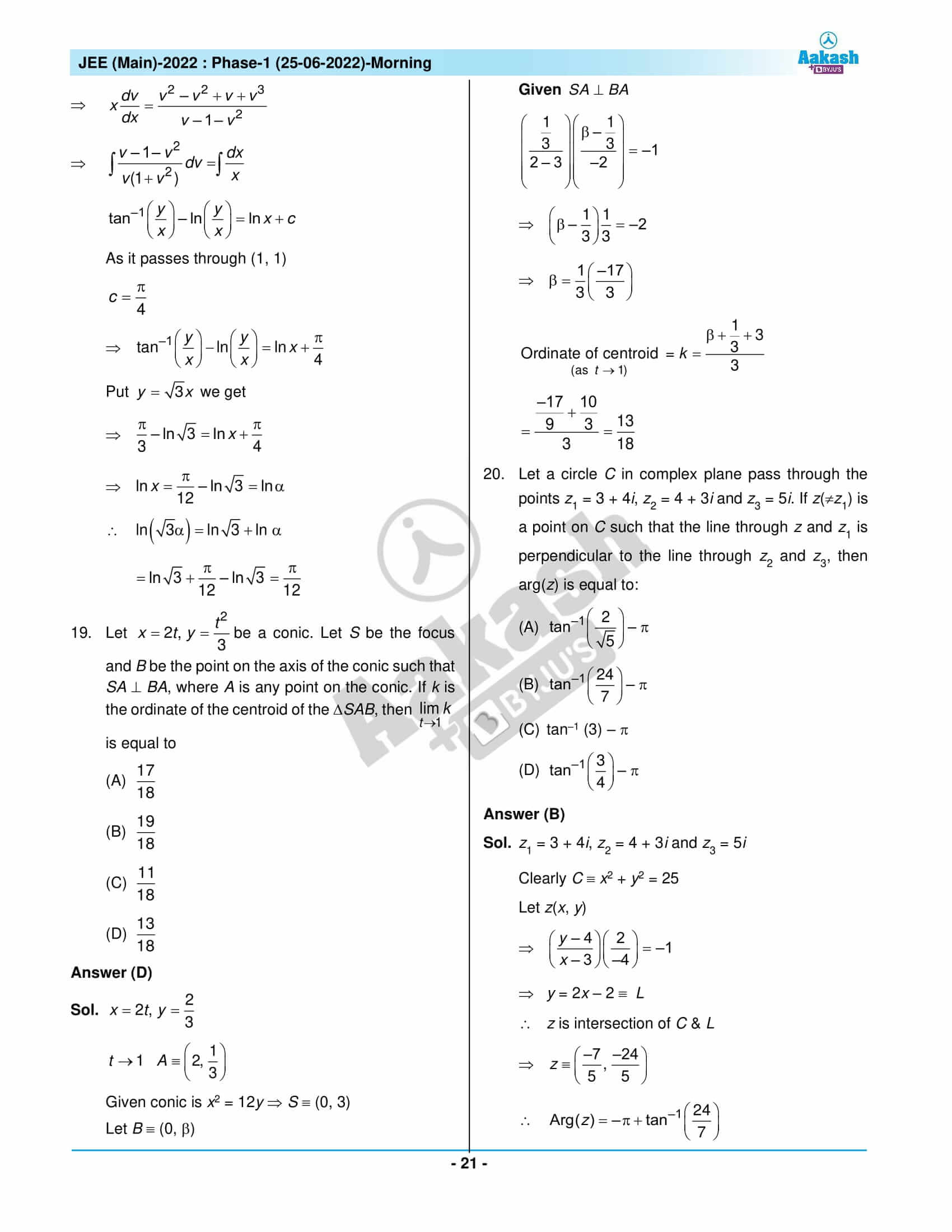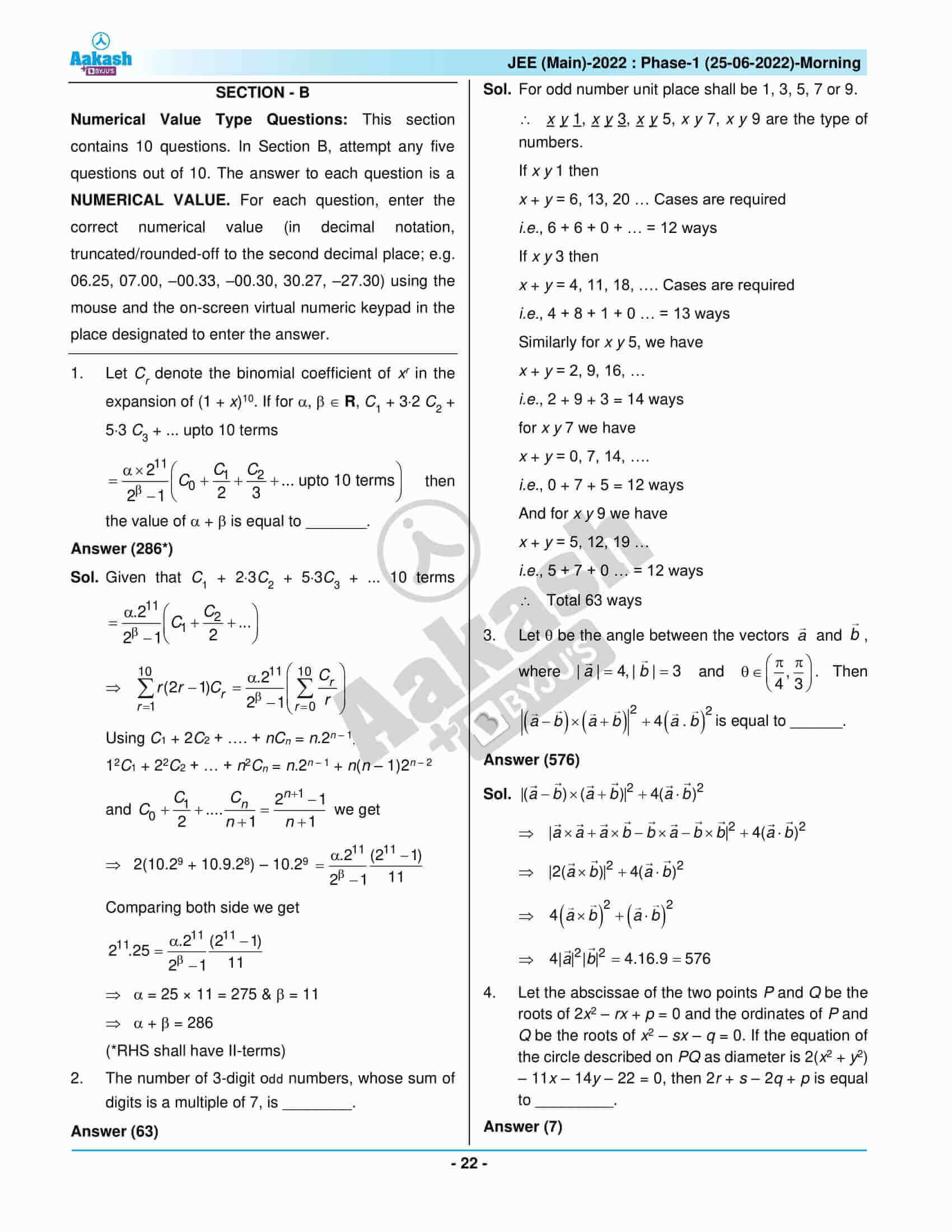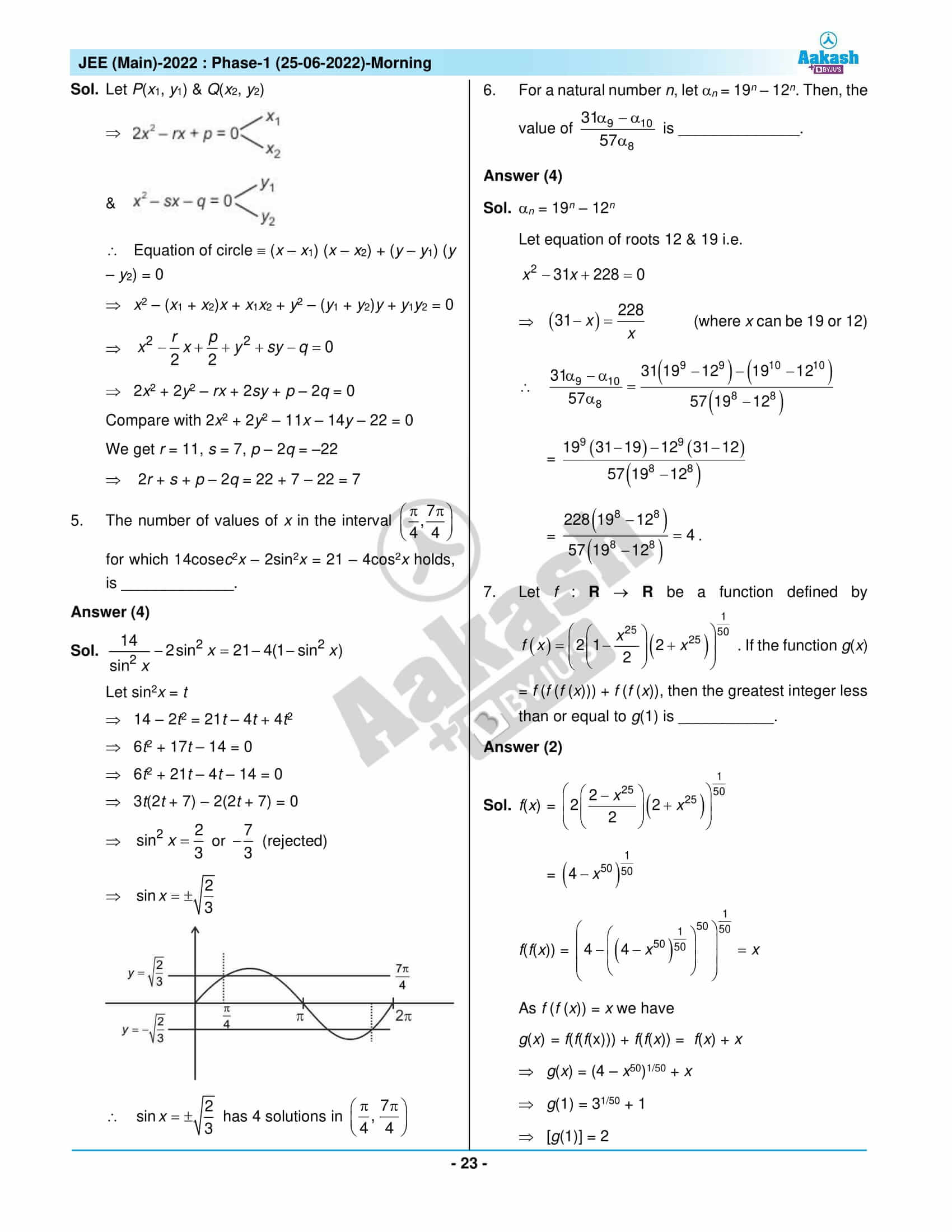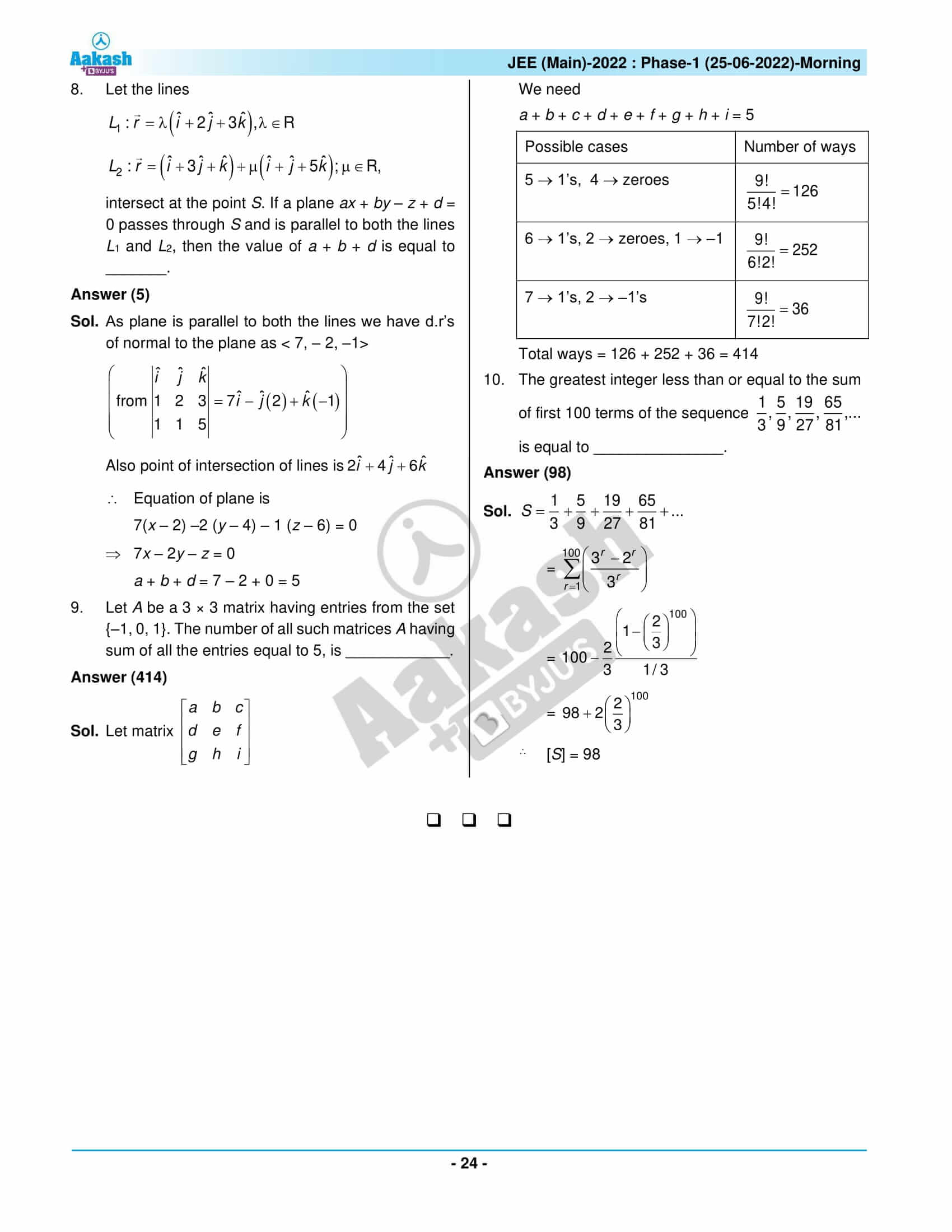Win up to 100% scholarship on Aakash BYJU'S JEE/NEET courses with ABNAT Win up to 100% scholarship on Aakash BYJU'S JEE/NEET courses with ABNAT

# JEE Main 2022 June 25 - Shift 1 Maths Question Paper with Solutions

The JEE Main 2022 June 25 – Shift 1 Maths Question Paper with Solutions is available on this page. The solutions for JEE Main 2022 question papers are prepared by an expert team at BYJU’S. The students can easily understand these step-by-step solutions of the JEE Main 2022 question paper. The PDF format of JEE Main 2022 June 25 – Shift 1 Maths Question Paper with Solutions can be downloaded for free. These solutions will help students evaluate their scores in the JEE Main 2022 June 25 – Shift 1 Maths exam.
JEE Main 2022 June 25 – Shift 1 Maths Question Paper with Solutions

SECTION – A

Multiple Choice Questions: This section contains 20 multiple choice questions. Each question has 4 choices (1), (2), (3) and (4), out of which ONLY ONE is correct.

1. Let a circle C touch the lines L1 : 4x – 3y +K1 = 0 and L2 : 4x – 3y + K2 = 0, K1, K2R. If a line passing through the centre of the circle C intersects L1 at (–1, 2) and L2 at (3, –6), then the equation of the circle Cis :

(A) (x – 1)2 + (y – 2)2 = 4

(B) (x + 1)2 + (y – 2)2 = 4

(C) (x – 1)2 + (y + 2)2 = 16

(D) (x – 1)2 + (y – 2)2 = 16

Sol.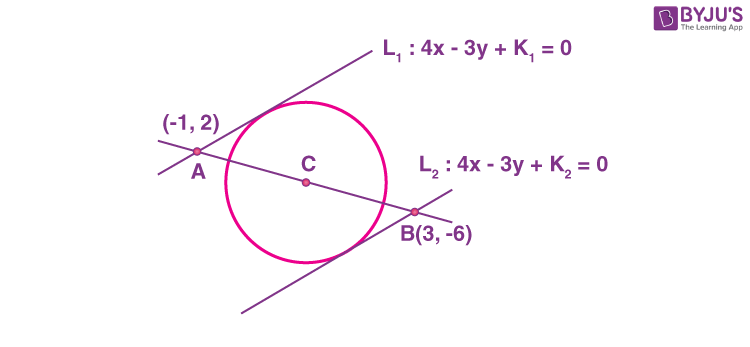Co-ordinate of centre

$$\begin{array}{l}C=\left ( \frac{3+\left ( -1 \right )}{2},\frac{-6+2}{2} \right )\equiv \left ( 1,-2 \right )\end{array}$$

L1 is passing through A

⇒ –4 – 6 + K1 = 0

K1 = 10

L2 is passing through B

⇒ 12 + 18 + K2 = 0

K2 = –30

Equation of L1 : 4x – 3y + 10 = 0

Equation of L1 : 4x – 3y – 30 = 0

Diameter of circle

$$\begin{array}{l}=\left|\frac{10+30}{\sqrt{4^2+\left ( -3 \right )^2}} \right|=8\end{array}$$

Equation of circle (x – 1)2 + (y + 2)2 = 16

2. The value of

$$\begin{array}{l}\displaystyle\int\limits_0^\pi\frac{e^{\cos x}\sin x}{\left ( 1+\cos^2x \right )\left ( e^{\cos x}+e^{-\cos x} \right )}dx\end{array}$$
is equal to :

(A)

$$\begin{array}{l}\frac{\pi^2}{4}\end{array}$$

(B)

$$\begin{array}{l}\frac{\pi^2}{2}\end{array}$$

(C)

$$\begin{array}{l}\frac{\pi}{4}\end{array}$$

(D)

$$\begin{array}{l}\frac{\pi}{2}\end{array}$$

Sol.

$$\begin{array}{l}\displaystyle\int\limits_0^\pi\frac{e^{\cos x}\sin x}{\left ( 1+\cos^2x \right )\left ( e^{\cos x}+e^{-\cos x} \right )}dx\end{array}$$

Let cosx = t

sinxdx = dt

$$\begin{array}{l}=\displaystyle\int \limits_1^{-1}\frac{-e^tdt}{\left ( 1+t^2 \right )\left ( e^t+e^{-t} \right )}\end{array}$$
$$\begin{array}{l}I=\displaystyle\int \limits_{-1}^1\frac{e^{-t}}{\left ( 1+t^2 \right )\left ( e^{t}+e^{-t} \right )}dt\dots\left ( i \right )\end{array}$$
$$\begin{array}{l}I=\displaystyle\int \limits_{-1}^1\frac{e^{-t}}{\left ( 1+t^2 \right )\left ( e^{-t}+e^t \right )}dt\dots\left ( ii \right )\end{array}$$

$$\begin{array}{l}2I=\displaystyle\int \limits_{-1}^1\frac{dt}{1+t^2}\end{array}$$
$$\begin{array}{l}\left.\begin{matrix}2I = \tan^{-t}\end{matrix}\right]_{-1}^{1}\end{array}$$
$$\begin{array}{l}2I=\frac{\pi}{4}-\left ( -\frac{\pi}{4} \right )\end{array}$$
$$\begin{array}{l}2I=\frac{\pi}{2}\end{array}$$
$$\begin{array}{l}I=\frac{\pi}{4}\end{array}$$

3. Let a, b and c be the length of sides of a triangle ABC such that

$$\begin{array}{l}\frac{a+b}{7}=\frac{b+c}{8}=\frac{c+a}{9}\end{array}$$
. If r and R are the radius of incircle and radius of circumcircle of the triangle ABC, respectively, then the value of
$$\begin{array}{l}\frac{R}{r}\end{array}$$
is equal to :

(A)

$$\begin{array}{l}\frac{5}{2}\end{array}$$

(B) 2

(C)

$$\begin{array}{l}\frac{3}{2}\end{array}$$

(D) 1

Sol.

$$\begin{array}{l}\frac{a+b}{7}=\frac{b+c}{8}=\frac{c+a}{9}=\lambda\end{array}$$
$$\begin{array}{l}\begin{array}{c} a + b = 7 \lambda\\ b + c = 8 \lambda\\c + a = 9 \lambda\\\hline a + b + c 12\lambda \\ \end{array}\end{array}$$

a = 4λ, b = 3λ, c = 5λ

$$\begin{array}{l}s=\frac{4\lambda+3\lambda+5\lambda}{2} =6\lambda\end{array}$$
$$\begin{array}{l}\Delta=\sqrt{s\left ( s-a \right )\left ( s-b \right )\left ( s-c \right )}=\sqrt{\left ( 6\lambda \right )\left ( 2\lambda \right )\left ( 3\lambda \right )\left ( \lambda \right )}\end{array}$$

= 6λ2

$$\begin{array}{l}R=\frac{abc}{4\Delta}=\frac{\left ( 4\lambda \right )\left ( 3\lambda \right )\left ( 5\lambda \right )}{4\left ( 6\lambda^2 \right )}= \frac{5}{2}\lambda\end{array}$$
$$\begin{array}{l}r=\frac{\Delta}{s}=\frac{6\lambda^2}{6\lambda}=\lambda\end{array}$$
$$\begin{array}{l}\frac{R}{r}=\frac{\frac{5}{2}\lambda}{\lambda}=\frac{5}{2}\end{array}$$

4. Let ƒ : NR be a function such that ƒ(x + y) = 2ƒ(x) ƒ(y) for natural numbers x and y. If ƒ(1) = 2, then the value of α for which

$$\begin{array}{l}\sum_{k=1}^{10}f\left ( \alpha+k \right )=\frac{512}{3}\left ( 2^{20}-1 \right )|\end{array}$$

holds, is :

(A) 2

(B) 3

(C) 4

(D) 6

Sol. ƒ(x + y) = 2ƒ(x)ƒ(y) & ƒ(1) = 2

x = y = 1

$$\begin{array}{l}\left.\begin{matrix}\Rightarrow f\left ( 2 \right )=2^3\\~~~~~~x=2,y=1\\\Rightarrow f\left ( 3)=2^5 \right )\end{matrix}\right\}\! f\left ( x \right )=2^{\left ( 2x-1 \right )}\end{array}$$

Now,

$$\begin{array}{l}\displaystyle\sum\limits_{k=1}^{10}f(\alpha+k)=\frac{512}{3}\left ( 2^{20}-1 \right ) \end{array}$$
$$\begin{array}{l}2\displaystyle\sum\limits_{k=1}^{10}f(\alpha)f\left ( k \right )=\frac{512}{3}\left ( 2^{20}-1 \right ) \end{array}$$
$$\begin{array}{l}2f\left ( \alpha \right )\left [ f\left ( 1 \right )+f\left ( 2 \right )+\cdots+f\left ( 10 \right ) \right ]=\frac{512}{3}(2^{20}-1)\end{array}$$
$$\begin{array}{l}2f\left ( \alpha \right )\left [ 2+2^3+2^5+\cdots \text{upto~10~terms} \right ]=\frac{512}{3}(2^{20}-1)\end{array}$$
$$\begin{array}{l}2f\left ( \alpha \right )\cdot2\left ( \frac{2^{20}-1}{4-1}\right )=\frac{512}{3}(2^{20}-1)\end{array}$$

ƒ(α) = 128 = 2 – 1

= 2α – 1 = 7

⇒ α = 4

5. Let A be a 3 × 3 real matrix such that

$$\begin{array}{l}A\begin{pmatrix}1 \\1 \\0\end{pmatrix}= \begin{pmatrix}1 \\1 \\0\end{pmatrix};~~A \begin{pmatrix}1 \\0 \\1\end{pmatrix}= \begin{pmatrix}-1 \\0 \\1\end{pmatrix}\end{array}$$
and
$$\begin{array}{l}A\begin{pmatrix}0 \\0 \\1\end{pmatrix}= \begin{pmatrix}1 \\1 \\2\end{pmatrix}\end{array}$$

If X = (x1, x2, x3)T and I is an identity matrix of order 3, then the system

$$\begin{array}{l}\left ( A-2I \right )X=\begin{pmatrix} 4\\ 1\\1\end{pmatrix}\end{array}$$
has :

(A) No solution

(B) Infinitely many solutions

(C) Unique solution

(D) Exactly two solutions

Sol. Let

$$\begin{array}{l}A=\begin{bmatrix}a & b & c \\d & e & f \\g & h & i \\\end{bmatrix}\end{array}$$
$$\begin{array}{l}A\begin{bmatrix}1 \\1 \\0\end{bmatrix}=\begin{bmatrix}1 \\1 \\0\end{bmatrix}\Rightarrow\begin{bmatrix} a& b & c \\d & 3 & f \\g & h & i \\\end{bmatrix}=\begin{bmatrix}1 \\1 \\0\end{bmatrix}\Rightarrow\begin{matrix}a + b =1 \\d + e =1 \\g +h =0 \\\end{matrix} \end{array}$$
$$\begin{array}{l}A\begin{bmatrix} 1\\ 0\\ 1\end{bmatrix}=\begin{bmatrix}-1 \\ 0\\1\end{bmatrix}\Rightarrow\begin{bmatrix} a& b& c \\ d& e& f \\ g& h& i \\\end{bmatrix}\begin{bmatrix} 1\\ 0\\1\end{bmatrix}=\begin{bmatrix} -1\\ 0\\1\end{bmatrix}\Rightarrow\begin{matrix}a+c=-1\\d+f=0\\g+i=1\end{matrix}\end{array}$$
$$\begin{array}{l}A\begin{bmatrix} 0\\ 0\\ 1\end{bmatrix}=\begin{bmatrix}1 \\1 \\2\end{bmatrix}\Rightarrow\begin{bmatrix} a& b& c \\ d& e& f \\ g& h& i \\\end{bmatrix}\begin{bmatrix} 0\\ 0\\1\end{bmatrix}=\begin{bmatrix} 1\\ 1\\2\end{bmatrix}\Rightarrow\begin{matrix}c=1\\f=1\\i=2\end{matrix}\end{array}$$

Solving will get

a = –2, b = 3, c = 1, d = –1, e = 2, f = 1, g = –1,
h = 1, i = 2

$$\begin{array}{l}A=\begin{bmatrix}-2 & 3 & 1 \\-1 & 2 & 1 \\-1 & 1 & 2 \\\end{bmatrix}\Rightarrow A=2I=\begin{bmatrix}-4 & 3 & 1 \\-1 & 0 & 1 \\-1 & 1 & 0 \\\end{bmatrix} \end{array}$$
$$\begin{array}{l}\left ( A-2I \right )x=\begin{bmatrix}4 \\1 \\1\end{bmatrix}\end{array}$$

⇒ –4x1 + 3x2 + x3 = 4 …(i)

x1 + x3 = 1 …(ii)

x1 + x2 = 1 …(iii)

So 3(iii) + (ii) = (i)

∴Infinite solution

6. Let ƒ : RR be defined as

ƒ(x) = x3 + x – 5

If g(x) is a function such that ƒ(g(x)) =

$$\begin{array}{l}x,\forall^{‘}x^{‘}\epsilon \textbf{R}\end{array}$$
then g′(63) is equal to_____.

(A)

$$\begin{array}{l}\frac{1}{49}\end{array}$$

(B)

$$\begin{array}{l}\frac{3}{49}\end{array}$$

(C)

$$\begin{array}{l}\frac{43}{49}\end{array}$$

(D)

$$\begin{array}{l}\frac{91}{49}\end{array}$$

Sol.ƒ(x) = 3x2 + 1

ƒ′(x) is bijective function

and ƒ(g(x)) = xg(x) is inverse of ƒ(x)

g(ƒ(x)) = x

g′(f(x)).f′(x) = 1

$$\begin{array}{l}g’\left ( f\left ( x \right ) \right )=\frac{1}{3x^2+1}\end{array}$$

Put x = 4 we get

$$\begin{array}{l}g’\left ( 63 \right )=\frac{1}{49}\end{array}$$

7. Consider the following two propositions :

P1 : ~ (p → ~ q)

P2: (p ~q) ((-~p) ∨ q)

If the proposition p → ((~p) q) is evaluated as FALSE, then :

(A) P1 is TRUE and P2 is FALSE

(B) P1 is FALSE and P2 is TRUE

(C) Both P1 and P2 are FALSE

(D) Both P1 and P2 are TRUE

Sol. Given p → (~ pq) is false

⇒ ~ p q is false and p is true

Now p = True.

~ T q = F

F q = Fq is false

P1 : ~ (T → ~ F) ≡ ~ (TT) ≡ False.

P2 : (T~ F) (~ T F) ≡ (TT) (FF)

TF ≡ False

8. If

$$\begin{array}{l}\frac{1}{2\cdot3^{10}}+\frac{1}{2^2\cdot3^9}+\dots+\frac{1}{2^{10}\cdot3}=\frac{K}{2^{10}\cdot3^{10}}\end{array}$$
then the remainder when K is divided by 6 is :

(A) 1

(B) 2

(C) 3

(D) 5

Sol.

$$\begin{array}{l}\frac{1}{2\cdot3^{10}}+\frac{1}{2^2\cdot3^9}+\dots+\frac{1}{2^{10}\cdot3}=\frac{K}{2^{10}\cdot3^{10}}\end{array}$$
$$\begin{array}{l}\frac{1}{2\cdot3^{10}}\left [ \frac{\left ( \frac{3}{2}^{10} \right )-1}{\frac{3}{2}-1} \right ]=\frac{K}{2^{10}\cdot3^{10}}\end{array}$$
$$\begin{array}{l}=\frac{3^{10}-2^{10}}{2^{10}\cdot3^{10}}=\frac{K}{2^{10}\cdot3^{10}}\Rightarrow K=3^{10}-2^{10}\end{array}$$

Now K = (1 + 2)10 – 210

= 10C0 + 10C1 2 + 10C2 23 + … + 10C10 210 – 210

= 10C0 + 10C1 2 + 6λ + 10C9⋅ 29

= 1 + 20 + 5120 + 6λ

= 5136 + 6λ + 5

= 6μ + 5

λ, μ ∈ N

∴ remainder = 5

9. Let ƒ(x) be a polynomial function such that ƒ(x) + ƒ′(x) + ƒ′′(x) = x5 + 64. Then, the value of

$$\begin{array}{l}\displaystyle \lim_{ x\to 1}\frac{f\left ( x \right )}{x-1}\end{array}$$
is equal to :

(A) –15 (B) –60

(C) 60 (D) 15

Sol.

$$\begin{array}{l}\displaystyle \lim_{ x\to 1}\frac{f\left ( x \right )}{x-1}\end{array}$$

ƒ(x) + ƒ′(x) + ƒ′′(x) = x5 + 64

Let ƒ(x) = x5 + ax4 + bx3 + cx2 + dx + e

ƒ′(x) = 5x4 + 4ax3 + 3bx2 + 2cx + d

ƒ′′(x) = 20x3 + 12ax2 + 6bx + 2c

x5 + (a + 5)x4 + (b + 4a + 20) x3 + (c + 3b + 12a) x2 + (d + 2c + 6b) x + e + d + 2c = x5 + 64

a + 5 = 0

b + 4a + 20 = 0

c + 3b + 12a = 0

d + 2c + 6b = 0

e + d + 2c = 64

a = – 5, b = 0, c = 60, d = –120,e = 64

ƒ(x) = x5 – 5x4 + 60x2 – 120x + 64

Now,

$$\begin{array}{l}\displaystyle \lim_{ x\to 1}\frac{x^5-5x^4+60x^2-120x+64}{x-1}~ \text{is}\left ( \frac{0}{0}~\textup{from} \right )\end{array}$$

By L′ Hopital rule

$$\begin{array}{l}\displaystyle \lim_{ x\to 1}\frac{5x^4-20x^3+120x-120}{1}\end{array}$$

= –15

10. Let E1 and E2 be two events such that the conditional probabilities

$$\begin{array}{l}P\left ( E_1|E_2 \right )=\frac{1}{2},\end{array}$$
$$\begin{array}{l}P\left ( E_2|E_1 \right )=\frac{3}{4}\end{array}$$
and
$$\begin{array}{l}P\left ( E_1\cap E_2 \right )=\frac{1}{8}\cdot\end{array}$$
Then:

(A)

$$\begin{array}{l}P\left ( E_1\cap E_2 \right )=P\left ( E_1 \right )\cdot P\left ( E_2 \right )\end{array}$$

(B)

$$\begin{array}{l}P\left ( E_1^{‘}\cap E_2^{‘} \right )=P\left ( E_1^{‘} \right )\cdot P\left ( E_2 \right )\end{array}$$

(C)

$$\begin{array}{l}P\left ( E_1\cap E_2^{‘} \right )=P\left ( E_1 \right )\cdot P\left ( E_2 \right )\end{array}$$

(D)

$$\begin{array}{l}P\left ( E_1^{‘}\cap E_2 \right )=P\left ( E_1 \right )\cdot P\left ( E_2 \right )\end{array}$$

Sol.

$$\begin{array}{l}P\left ( \frac{E_1}{E_2} \right )=\frac{1}{2}\Rightarrow\frac{P\left ( E_1\cap E_2 \right )}{P\left ( E_2 \right )}=\frac{1}{2}\end{array}$$
$$\begin{array}{l}P\left ( \frac{E_2}{E_1} \right )=\frac{3}{4}\Rightarrow\frac{P\left ( E_2\cap E_1 \right )}{P\left ( E_1 \right )}=\frac{3}{4}\end{array}$$
$$\begin{array}{l}P\left ( E_1\cap E_2 \right )=\frac{1}{8}\end{array}$$
$$\begin{array}{l}P\left ( E_2 \right )=\frac{1}{4},P\left ( E_1 \right )=\frac{1}{6}\end{array}$$

(A)

$$\begin{array}{l}P\left ( E_1\cap E_2 \right )=\frac{1}{8}~\text{and}~P\left ( E_1 \right )\cdot P\left ( E_2 \right )=\frac{1}{24}\end{array}$$

$$\begin{array}{l}P\left ( E_1\cap E_2 \right )\neq P\left (E_1 \right ).P\left ( E_2 \right )\end{array}$$

(B)

$$\begin{array}{l}P\left ( E_1^{‘}\cap E_2^{‘} \right )=1-P\left ( E_1\cup E_2 \right )\end{array}$$
$$\begin{array}{l}=1-\left [ \frac{1}{4}+\frac{1}{6}-\frac{1}{8} \right ]=\frac{17}{24}\end{array}$$
$$\begin{array}{l}P\left ( E_1^{‘} \right )=\frac{3}{4}\Rightarrow P\left ( E_1^{‘} \right )P\left ( E_2 \right )=\frac{3}{24}\end{array}$$

$$\begin{array}{l}P\left ( E_1^{‘}\cap E_2^{‘} \right )\neq P\left ( E_1^{‘} \right )\cdot P\left ( E_2 \right )\end{array}$$

(C)

$$\begin{array}{l}P\left ( E_1\cap E_2^{‘} \right )= P\left ( E_1 \right )- P\left (E_1\cap E_2 \right )\end{array}$$
$$\begin{array}{l}=\frac{1}{6}-\frac{1}{8}=\frac{1}{24}\end{array}$$
$$\begin{array}{l}P\left ( E_1 \right )\cdot P\left ( E_2 \right )=\frac{1}{24}\end{array}$$

$$\begin{array}{l}P\left ( E_1\cap E_2^{‘} \right )=P\left ( E_1 \right )\cdot P\left ( E_2 \right )\end{array}$$

(D)

$$\begin{array}{l}P\left ( E_1^{‘}\cap E_2 \right )=P\left ( E_2 \right )-P\left ( E_1\cap E_2 \right )\end{array}$$
$$\begin{array}{l}=\frac{1}{4}-\frac{1}{8}=\frac{1}{8}\end{array}$$
$$\begin{array}{l}P\left ( E_1 \right )P\left ( E_2 \right )=\frac{1}{24}\end{array}$$

$$\begin{array}{l}P\left ( E_1^{‘}\cap E_2 \right )\neq P\left ( E_1 \right ).P\left ( E_2 \right )\end{array}$$

11. Let

$$\begin{array}{l}A=\begin{bmatrix}0 & -2 \\2 & 0 \\\end{bmatrix}\end{array}$$
If M and N are two matrices given by
$$\begin{array}{l}M=\displaystyle\sum\limits_{k=1}^{10} A^{2k}\end{array}$$
and
$$\begin{array}{l}N=\displaystyle\sum\limits_{k=1}^{10}A^{2k-1}\end{array}$$
then MN2is :

(A) a non-identity symmetric matrix

(B) a skew-symmetric matrix

(C) neither symmetric nor skew-symmetric matrix

(D) an identity matrix

Sol.

$$\begin{array}{l}A=\begin{bmatrix}0 & -2 \\2 & 0 \\\end{bmatrix}\end{array}$$
$$\begin{array}{l}A^2=\begin{bmatrix}0 & -2 \\2 & 0 \\\end{bmatrix}\begin{bmatrix}0 & -2 \\2 & 0 \\\end{bmatrix}=\begin{bmatrix}-4 & 0 \\0 & -4 \\\end{bmatrix}=-4I \end{array}$$

M = A2 + A4 + A6 + … + A20

= –4I + 16l – 64I + … upto 10 terms

= –I [4 – 16 + 64 … + upto 10 terms]

$$\begin{array}{l}=-I\cdot 4\left [ \frac{\left ( -4 \right )^{10}-1}{-4-1} \right ]=\frac{4}{5}\left ( 2^{20}-1 \right )I\end{array}$$

= A – 4A + 16A + … upto 10 terms

$$\begin{array}{l}=A\left ( \frac{\left ( -4 \right )^{10}-1}{-4-1} \right )=- \left ( \frac{2^{20}-1}{5} \right )A\end{array}$$
$$\begin{array}{l}N^2=\frac{(2^{20}-1)^2}{2^5}A^2=\frac{-4}{25}\left ( 2^{20}-1 \right )^2t\end{array}$$
$$\begin{array}{l}MN^2=\frac{-16}{125}\left ( 2^{20}-1 \right )^3I=KI~~~~\left ( K\neq\pm 1 \right )\end{array}$$

(MN2)T = (KI)T = KI

∴ A is correct

12. Let g : (0, ∞) →R be a differentiable function such that

$$\begin{array}{l}\int\left ( \frac{x\left ( \cos x-\sin x \right )}{e^x+1}+\frac{g\left ( x \right )\left ( e^x+1-xe^x \right )}{\left ( e^x+1 \right )^2} \right ) dx=\frac{xg\left ( x \right )}{e^x+1}+c,\end{array}$$
for all x> 0, where c is an arbitrary constant. Then :

(A) g is decreasingin

$$\begin{array}{l}\left ( 0, \frac{\pi}{4} \right )\end{array}$$

(B) g′ is increasing in

$$\begin{array}{l}\left ( 0, \frac{\pi}{4} \right )\end{array}$$

(C) g + g′ is increasing in

$$\begin{array}{l}\left ( 0, \frac{\pi}{2} \right )\end{array}$$

(D) gg′ is increasing in

$$\begin{array}{l}\left ( 0, \frac{\pi}{2} \right )\end{array}$$

Sol.

$$\begin{array}{l}\int\left ( \frac{x\left ( \cos x-\sin x \right )}{e^x+1}+\frac{g\left ( x \right )\left ( e^x+1-xe^x \right )}{\left ( e^x+1 \right )^2} \right ) dx=\frac{xg\left ( x \right )}{e^x+1}+c\end{array}$$

Differentiating on both sides

$$\begin{array}{l}\frac{x\left ( \cos x-\sin x \right )}{e^x+1}+\frac{g\left ( x \right )\left ( e^x+1-xe^x \right )}{\left ( e^x-1 \right )^2}\end{array}$$
$$\begin{array}{l}=\frac{\left ( e^x+1 \right )\left ( g\left ( x \right )+xg^\frac{1}{x} \right )-xg\left ( x \right )e^x}{\left ( e^x+1 \right )^2}\end{array}$$
$$\begin{array}{l}=\frac{g\left ( x \right )\left [ e^x+1-xe^x \right ]}{\left ( e^x+1 \right )^2}+\frac{xg^{‘}\left ( x \right )\left ( e^x+1 \right )}{\left ( e^x+1 \right )^2}\end{array}$$
$$\begin{array}{l}=\frac{x\left ( \cos x-\sin x \right )}{e^x+1}=\frac{xg^{‘}\left ( x \right )}{e^x+1}\end{array}$$

$$\begin{array}{l}g^{‘}\left ( x \right )=\cos x-\sin x > 0~\textup{in}\left ( 0,\frac{\pi}{4} \right )\end{array}$$

⇒ g(x) is increasing in

$$\begin{array}{l}\left ( 0,\frac{\pi}{4} \right )\end{array}$$
⇒A is wrong

Now,

$$\begin{array}{l}g^{”}\left ( x \right )=-\sin x -\cos x<0~\textup{in}~\left ( 0,\frac{\pi}{4} \right )\end{array}$$

g(x) is increasing in

$$\begin{array}{l}\left ( 0,\frac{\pi}{4} \right )\end{array}$$
B is wrong

Let h(x) = g(x) + g′(x)

$$\begin{array}{l}h^{‘}\left ( x \right )=g^{‘}\left ( x \right )+g^{”}\left ( x \right )=-2\sin x < 0~\textup{in}~x\epsilon\left ( 0,\frac{\pi}{2} \right )\end{array}$$

g + g′is decreasing in

$$\begin{array}{l}\left ( 0,\frac{\pi}{2} \right )\end{array}$$

c is wrong

Let J(x) = g(x) – g′(x)

$$\begin{array}{l}J^{‘}\left ( x \right )=g^{‘}\left ( x \right )-g^{”}\left ( x \right )=2\cos x>0~\text{in}~\left ( 0,\frac{\pi}{2} \right )\end{array}$$

gg′ is increasing in

$$\begin{array}{l}\left ( 0,\frac{\pi}{2} \right )\Rightarrow~\textup{correct}\end{array}$$

13. Let f :RR and g : RR be two functions defined by f(x) = loge(x2 + 1) – ex + 1 and

$$\begin{array}{l}g\left ( x \right )=\frac{1-2e^{2x}}{e^x}\end{array}$$
. Then, for which of the following range of α, the inequality
$$\begin{array}{l}f\left ( g\left ( \frac{\left ( \alpha-1 \right )^2}{3} \right ) \right )>f\left ( g\left ( \alpha-\frac{5}{3} \right ) \right )\end{array}$$
holds?

(A) (2, 3)

(B) (–2, –1)

(C) (1, 2)

(D) (–1, 1)

Sol.

$$\begin{array}{l}f\left ( x \right )=log_e\left ( x^2+1 \right )-e^{-x}+1\end{array}$$
$$\begin{array}{l}f^{‘}\left ( x \right )=\frac{2x}{x^2+1}+e^{-x}\end{array}$$
$$\begin{array}{l}=\frac{2}{x+\frac{1}{x}}+e^{-x}>0~~\forall x\epsilon R\end{array}$$
$$\begin{array}{l}g\left ( x \right )=e^{-x}-2e^x\end{array}$$
$$\begin{array}{l}g^{‘}\left ( x \right )=-e^{-x}-2e^x<0~~~\forall x\epsilon R\end{array}$$

f(x) is increasing and g(x) is decreasing function.

$$\begin{array}{l}f\left ( g\left ( \frac{\left ( \alpha-1 \right )^2}{3} \right ) \right )>f\left ( g\left ( \alpha-\frac{5}{3} \right ) \right )\end{array}$$
$$\begin{array}{l}\Rightarrow\frac{\left ( \alpha-1 \right )^2}{3}<\alpha-\frac{5}{3}\end{array}$$

= α2 – 5α + 6 < 0

= (α – 2)(α – 3) < 0

= α∈(2, 3)

14. Let

$$\begin{array}{l}\vec{a}=a_1\hat{i}+a_2\hat{j}+a_3\hat{k}a_i>0, i=1,~2,~3\end{array}$$
be a vector which makes equal angles with the coordinate axes OX, OY and OZ. Also, let the projection of
$$\begin{array}{l}\vec{a}\end{array}$$
on the vector
$$\begin{array}{l}3\hat{i}+4\hat{j}\end{array}$$
be 7. Let
$$\begin{array}{l}\vec{b}\end{array}$$
be a vector obtained by rotating
$$\begin{array}{l}\vec{a}\end{array}$$
with 90°. If
$$\begin{array}{l}\vec{a},\vec{b}\end{array}$$
and x-axis are coplanar, then projection of a vector
$$\begin{array}{l}\vec{b}\end{array}$$
on
$$\begin{array}{l}3\hat{i}+4\hat{j}\end{array}$$
is equal to :

(A)

$$\begin{array}{l}\sqrt{7}\end{array}$$

(B)

$$\begin{array}{l}\sqrt{2}\end{array}$$

(C) 2

(D) 7

Sol.

$$\begin{array}{l}\cos^2~\alpha+cos^2~\beta+\cos^2~\gamma=1~\Rightarrow~\cos^2~\alpha=\frac{1}{3}\end{array}$$
$$\begin{array}{l}\Rightarrow\cos\alpha=\frac{1}{\sqrt{3}}\end{array}$$
$$\begin{array}{l}\vec{a}=\frac{\lambda}{3}\left ( \hat{i}+\hat{j}+\hat{k} \right ),\lambda>0\end{array}$$
$$\begin{array}{l}\frac{\lambda}{\sqrt{3}}\frac{\left ( \hat{i}+\hat{j}+\hat{k} \right )\cdot\left ( 3\hat{i}+4\hat{j} \right )}{\sqrt{3^2+4^2}}=7\end{array}$$
$$\begin{array}{l}\Rightarrow\frac{\lambda}{\sqrt{3}}\left ( 3+4 \right )=7\times5\end{array}$$

$$\begin{array}{l}\lambda=5\sqrt{3}\end{array}$$
$$\begin{array}{l}\vec{a}=5\left ( \hat{i}+\hat{j}+\hat{k} \right )\end{array}$$

Let

$$\begin{array}{l}\vec{b}=p\hat{i}+q\hat{j}+r\hat{k}\end{array}$$
$$\begin{array}{l}\vec{a}\cdot\vec{b}=0~\text{and}~\left [ \vec{a}~\vec{b}~\hat{i} \right ]=0\end{array}$$

p + q + r = 0 …(i)

&

$$\begin{array}{l}\begin{vmatrix}p & q & r \\1 & 1 & 1 \\1 & 0 & 0 \\\end{vmatrix}=0\end{array}$$
$$\begin{array}{l}\Rightarrow\vec{b}=-2r\hat{i}+r\hat{j}+r\hat{k}[\end{array}$$
$$\begin{array}{l}\vec{b}=r\left ( -2\hat{i}+\hat{j}+\hat{k} \right )\end{array}$$

Now

$$\begin{array}{l}\left|\vec{a} \right|=\left|\vec{b} \right|\end{array}$$
$$\begin{array}{l}5\sqrt{3}=\left|r \right|\sqrt{b}\Rightarrow~\left|r \right|=\frac{5}{\sqrt{2}}\end{array}$$

⇒ Projection of

$$\begin{array}{l}\vec{b}\end{array}$$
on
$$\begin{array}{l}3\hat{i}+4\hat{j}=\left|\frac{\vec{b}\cdot\left ( 3\hat{i}+4\hat{j} \right )}{\sqrt{3^2+4^2}} \right|\end{array}$$
$$\begin{array}{l}=\left|r \right|\frac{\left ( -6+4 \right )}{5}=\left|\frac{-2}{5} \right|\end{array}$$

Projection

$$\begin{array}{l}=\frac{2}{5}\times\frac{5}{\sqrt{2}}=\sqrt{2}\end{array}$$

∴ B is correct

15. Let y = y(x) be the solution of the differential equation (x + 1)y′ – y = e3x(x + 1)2, with

$$\begin{array}{l}y\left ( 0 \right )=\frac{1}{3}\end{array}$$
Then, the point
$$\begin{array}{l}x=-\frac{4}{3}\end{array}$$
for the curve y = y(x)is :

(A) not a critical point

(B) a point of local minima

(C) a point of local maxima

(D) a point of inflection

Sol.

$$\begin{array}{l}\left ( x+1 \right )\frac{dy}{dx}-y=e^{3x}\left ( x+1 \right )^2\end{array}$$
$$\begin{array}{l}\frac{dy}{dx}-\frac{y}{x+1}=e^{3x}\left ( x+1 \right )\end{array}$$

If

$$\begin{array}{l}e^{-\int\frac{1}{x+1}x }=e^{-log\left ( x+1 \right )}=\frac{1}{x+1}\end{array}$$

$$\begin{array}{l}y\left ( \frac{1}{x+1} \right )=\int\frac{e^{3x}\left ( x+1 \right )}{x+1}dx\end{array}$$
$$\begin{array}{l}\frac{y}{x+1}=\int e^{3x}dx\end{array}$$
$$\begin{array}{l}\frac{y}{x+1}=\frac{e^{3x}}{3}+c \end{array}$$
$$\begin{array}{l}\because y\left ( 0 \right )=\frac{1}{3}\end{array}$$
$$\begin{array}{l}\frac{1}{3}=\frac{1}{3}+c\end{array}$$
• c = 0

So,

$$\begin{array}{l}y=\frac{e^{3x}}{3}(x+1) \end{array}$$

$$\begin{array}{l}y^{‘}=e^{3x}\left ( x+1 \right )+\frac{e^{3x}}{3}=e^{3x}\left ( x+\frac{4}{3} \right )\end{array}$$
$$\begin{array}{l}y^{”}=3e^{3x}\left ( x+\frac{4}{3} \right )+e^{3x}=e^{3x}\left ( 3x+5 \right )\end{array}$$
$$\begin{array}{l}y^{‘}=0~\textup{at}~x=\frac{-4}{3}~\&~y^{”}=e^{-4}\left ( 1 \right )>0~\textup{at}~x=\frac{-4}{3}\end{array}$$

$$\begin{array}{l}x=\frac{-4}{3}\end{array}$$
is point of local minima

16. If y = m1x + c1 and y = m2x + c2, m1m2 are two common tangents of circle x2 + y2 = 2 and parabola y2 = x, then the value of 8|m1m2| is equal to :

(A)

$$\begin{array}{l}3+4\sqrt{2}\end{array}$$

(B)

$$\begin{array}{l}-5+6\sqrt{2}\end{array}$$

(C)

$$\begin{array}{l}-4+3\sqrt{2}\end{array}$$

(D)

$$\begin{array}{l}7+6\sqrt{2}\end{array}$$

Sol. Let tangent to y2 = x be

$$\begin{array}{l}y=mx+\frac{1}{4m}\end{array}$$

For it being tangent to circle.

$$\begin{array}{l}\left|\frac{\frac{1}{4}m}{\sqrt{1+m^2}} \right|=\sqrt{2}\end{array}$$

⇒ 32m4 + 32m2 – 1 = 0

$$\begin{array}{l}m^2=\frac{-32\pm\sqrt{\left ( 32 \right )^2+4\left ( 32 \right )}}{64}\end{array}$$

$$\begin{array}{l}8m_1m_2=-4+3\sqrt{2}\end{array}$$

17. Let Q be the mirror image of the point P(1, 0, 1) with respect to the plane S: x + y + z = 5. If a line L passing through (1, –1, –1), parallel to the line PQ meets the plane S at R, then QR2 is equal to :

(A) 2 (B) 5

(C) 7 (D) 11

Sol. As L is parallel to PQ d.r.s of S is <1, 1, 1>

$$\begin{array}{l}L\equiv \frac{x-1}{1}=\frac{y+1}{1}=\frac{z+1}{1}\end{array}$$

Point of intersection of L and S be λ

⇒ (λ + 1) + (λ – 1) + (λ – 1) = S

⇒ λ = 2

R≡ (3, 1, 1)

Let Q(α, β, γ)

$$\begin{array}{l}\frac{\alpha-1}{1}=\frac{\beta}{1}=\frac{\gamma-1}{1}=\frac{-2\left ( 3 \right )}{3}\end{array}$$

⇒ α = 3, β = 2, γ = 3

Q≡ (3, 2, 3)

(QR)2 = 02 + (1)2 + (2)2 = 5

18. If the solution curve y = y(x) of the differential equation y2dx + (x2xy + y2)dy = 0, which passes through the point (1,1) and intersects the line

$$\begin{array}{l}y=\sqrt{3}x\end{array}$$
at the point
$$\begin{array}{l}\left ( \alpha,\sqrt{3}\alpha \right )\end{array}$$
, then value of
$$\begin{array}{l}log_e\left ( \sqrt{3}\alpha \right )\end{array}$$
is equal to :

(A)

$$\begin{array}{l}\frac{\pi}{3}\end{array}$$

(B)

$$\begin{array}{l}\frac{\pi}{2}\end{array}$$

(C)

$$\begin{array}{l}\frac{\pi}{12}\end{array}$$

(D)

$$\begin{array}{l}\frac{\pi}{6}\end{array}$$

Sol.

$$\begin{array}{l}\frac{dy}{dx}=\frac{y^2}{xy-x^2-y^2}\end{array}$$

Put y = vx we get

$$\begin{array}{l}v+x\frac{dv}{dx}=\frac{v^2}{v-1-v^2}\end{array}$$

$$\begin{array}{l}x\frac{dv}{dx}=\frac{v^2-v^2+v+v^3}{v-1-v^2}\end{array}$$

$$\begin{array}{l}\int\frac{v-1-v^2}{v\left ( 1+v^2 \right )}dv=\int\frac{dx}{x}\end{array}$$
$$\begin{array}{l}\tan^{-1}\left ( \frac{y}{x} \right )-\textup{In}\left ( \frac{y}{x} \right )=\textup{In}~x+c\end{array}$$

As it passes through (1, 1)

$$\begin{array}{l}c=\frac{\pi}{4}\end{array}$$

$$\begin{array}{l}\tan^{-1}\left ( \frac{y}{x} \right )-\textup{In}\left ( \frac{y}{x} \right )=\textup{In}~x+\frac{\pi}{4}\end{array}$$

Put

$$\begin{array}{l}y=\sqrt{3}x\end{array}$$
we get

$$\begin{array}{l}\frac{\pi}{3}-\textup{In}\sqrt{3}=\textup{In}~x+\frac{\pi}{4}\end{array}$$

$$\begin{array}{l}\textup{In}~x=\frac{\pi}{12}-\textup{In}\sqrt{3}=\textup{In}~\alpha\end{array}$$

$$\begin{array}{l}\textup{In}\left ( \sqrt{3}\alpha \right )=\textup{In}\sqrt{3}+\textup{In}~\alpha\end{array}$$
$$\begin{array}{l}=\textup{In}\sqrt{3}+\frac{\pi}{12}-\textup{In}\sqrt{3}=\frac{\pi}{12}\end{array}$$

19. Let

$$\begin{array}{l}x=2t,y=\frac{t^2}{3}\end{array}$$
be a conic. Let S be the focus and B be the point on the axis of the conic such that SABA, where A is any point on the conic. If k is the ordinate of the centroid of the ΔSAB, then
$$\begin{array}{l}\displaystyle \lim_{ t\to 1}k\end{array}$$
is equal to

(A)

$$\begin{array}{l}\frac{17}{18}\end{array}$$

(B)

$$\begin{array}{l}\frac{19}{18}\end{array}$$

(C)

$$\begin{array}{l}\frac{11}{18}\end{array}$$

(D)

$$\begin{array}{l}\frac{13}{18}\end{array}$$

Sol.

$$\begin{array}{l}x=2t,y=\frac{2}{3}\end{array}$$
$$\begin{array}{l}t\rightarrow1~~A\equiv\left ( 2,\frac{1}{3} \right )\end{array}$$

Given conic is x2 = 12y S ≡ (0, 3)

Let B ≡ (0, β)

GivenSABA

$$\begin{array}{l}\left ( \frac{\frac{1}{3}}{2-3} \right ) \left ( \frac{\beta-\frac{1}{3}}{-2} \right )=-1\end{array}$$

$$\begin{array}{l}\left ( \beta-\frac{1}{3} \right )\frac{1}{3}=-2\end{array}$$

$$\begin{array}{l}\beta=\frac{1}{3}\left ( \frac{-17}{3} \right )\end{array}$$
$$\begin{array}{l}\underset{\left ( \textup{as}~t\rightarrow1 \right )}{\textup{Ordinate~of~centroid}}=k=\frac{\beta+\frac{1}{3}+3}{3}\end{array}$$
$$\begin{array}{l}=\frac{\frac{-17}{9}+\frac{10}{3}}{3}=\frac{13}{18}\end{array}$$

20. Let a circle C in complex plane pass through the points z1 = 3 + 4i, z2 = 4 + 3i and z3 = 5i. If z(≠z1) is a point on C such that the line through z and z1 is perpendicular to the line through z2 and z3, then arg(z) is equal to:

(A)

$$\begin{array}{l}\tan^{-1}\left (\frac{2}{\sqrt{5}} \right )-\pi\end{array}$$

(B)

$$\begin{array}{l}\tan^{-1}\left ( \frac{24}{7} \right )-\pi\end{array}$$

(C) tan–1 (3) – π

(D)

$$\begin{array}{l}\tan^{-1}\left (\frac{3}{4} \right )-\pi\end{array}$$

Sol. z1 = 3 + 4i, z2 = 4 + 3i and z3 = 5i

Clearly C x2 + y2 = 25

Let z(x, y)

$$\begin{array}{l}\left ( \frac{y-4}{x-3}\right )\left ( \frac{2}{-4} \right )=-1\end{array}$$

y = 2x – 2 ≡ L

z is intersection of C&L

$$\begin{array}{l}z\equiv\left ( \frac{-7}{5},\frac{-24}{5} \right )\end{array}$$

$$\begin{array}{l}\textup{Arg}\left ( z \right )=-\pi+\tan^{-1}\left ( \frac{24}{7} \right )\end{array}$$

SECTION – B

Numerical Value Type Questions: This section contains 10 questions. In Section B, attempt any five questions out of 10. The answer to each question is a NUMERICAL VALUE. For each question, enter the correct numerical value (in decimal notation, truncated/rounded-off to the second decimal place; e.g. 06.25, 07.00, –00.33, –00.30, 30.27, –27.30) using the mouse and the on-screen virtual numeric keypad in the place designated to enter the answer.

1. Let Cr denote the binomial coefficient of xr in the expansion of (1 + x)10. If for α, β∈R, C1 + 3⋅2 C2 + 5⋅3 C3 + … upto 10 terms

$$\begin{array}{l}=\frac{\alpha\times2^{11}}{2^\beta-1}\left ( C_0+\frac{C_1}{2}+\frac{C_2}{3}+\dots\textup{upto~10~terms} \right )\end{array}$$
then the value of α + β is equal to _______.

Sol. Given that C1 + 2⋅3C2 + 5⋅3C3 + … 10 terms

$$\begin{array}{l}=\frac{\alpha\cdot2^{11}}{2^\beta-1}\left ( C_1+\frac{C_2}{2}+\dots \right )\end{array}$$

$$\begin{array}{l}\displaystyle\sum\limits_{r=1}^{10}r\left ( 2r-1 \right )C_r=\frac{\alpha\cdot 2^{11}}{2^\beta-1}\left ( \displaystyle\sum\limits_{r=0}^{10}\frac{C_r}{r} \right )\end{array}$$

Using C1 + 2C2 + …. + nCn = n.2n – 1,

12C1 + 22C2 + … + n2Cn = n.2n – 1 + n(n – 1)2n – 2

and

$$\begin{array}{l}C_0+\frac{C_1}{2}+\dots\frac{C_n}{n+1}=\frac{2^{n+1}-1}{n+1}\end{array}$$
we get

⇒ 2(10.29 + 10.9.28) – 10.29

$$\begin{array}{l}=\frac{\alpha2^{11}}{2^\beta-1} \frac{\left ( 2^{11}-1 \right )}{11}\end{array}$$

Comparing both side we get

$$\begin{array}{l}2^{11}.25=\frac{\alpha.2^{11}}{2^\beta-1}\frac{\left ( 2^{11}-1 \right )}{11}\end{array}$$

⇒ α = 25 × 11 = 275 &β = 11

⇒ α + β = 286

(*RHS shall have II-terms)

2. The number of 3-digit odd numbers, whose sum of digits is a multiple of 7, is ________.

Sol. For odd number unit place shall be 1, 3, 5, 7 or 9.

xy1, xy3, xy 5, xy 7, xy 9 are the type of numbers.

If xy 1 then

x + y = 6, 13, 20 … Cases are required

i.e., 6 + 6 + 0 + … = 12 ways

If xy 3 then

x + y = 4, 11, 18, …. Cases are required

i.e., 4 + 8 + 1 + 0 … = 13 ways

Similarly for xy 5, we have

x + y = 2, 9, 16, …

i.e., 2 + 9 + 3 = 14 ways

for xy 7 we have

x + y = 0, 7, 14, ….

i.e., 0 + 7 + 5 = 12 ways

And for xy 9 we have

x + y = 5, 12, 19 …

i.e., 5 + 7 + 0 … = 12 ways

∴ Total 63 ways

3. Let θ be the angle between the vectors

$$\begin{array}{l}\vec{a}\end{array}$$
and
$$\begin{array}{l}\vec{b}\end{array}$$
, where
$$\begin{array}{l}\left|\vec{a} \right|=4,\left|\vec{b} \right|=3\end{array}$$
and
$$\begin{array}{l}\theta\epsilon\left ( \frac{\pi}{4},\frac{\pi}{3} \right )\end{array}$$
. Then
$$\begin{array}{l}\left|\left ( \vec{a}-\vec{b} \right )\times\left ( \vec{a}+\vec{b} \right ) \right|^2+4\left ( \vec{a}.\vec{b} \right )^2\end{array}$$
is equal to ______.

Sol.

$$\begin{array}{l}\left|\left ( \vec{a}-\vec{b} \right )\times\left ( \vec{a}+\vec{b} \right ) \right|^2+4\left ( \vec{a}\cdot\vec{b} \right )^2\end{array}$$

$$\begin{array}{l}\left|\vec{a}\times\vec{a}+\vec{a}\times\vec{b}-\vec{b}\times\vec{a}-\vec{b}\times\vec{b} \right|^2+4\left ( \vec{a}\cdot\vec{b} \right )^2\end{array}$$

$$\begin{array}{l}\left|2\left ( \vec{a}\times\vec{b} \right ) \right|^2+4\left ( \vec{a}\cdot\vec{b} \right )^2\end{array}$$

$$\begin{array}{l}4\left ( \vec{a}\times\vec{b} \right )^2+\left ( \vec{a}\cdot\vec{b} \right )^2\end{array}$$

$$\begin{array}{l}4\left|\vec{a} \right|^2\left|\vec{b} \right|^2=4.16.9=576\end{array}$$

4. Let the abscissae of the two points P and Q be the roots of 2x2rx + p = 0 and the ordinates of P and Q be the roots of x2sxq = 0. If the equation of the circle described on PQ as diameter is 2(x2 + y2) – 11x – 14y – 22 = 0, then 2r + s – 2q + p is equal to _________.

Sol. Let P(x1, y1) &Q(x2, y2)

Solve 2x2rx + p = 0 for x1 and x2

Solve x2sxq = 0 for y1 and y2

∴ Equation of circle ≡ (xx1) (xx2) + (yy1) (yy2) = 0

x2 – (x1 + x2)x + x1x2 + y2 – (y1 + y2)y + y1y2 = 0

$$\begin{array}{l}x^2-\frac{r}{2}x+\frac{p}{2}+y^2+sy-q=0\end{array}$$

⇒ 2x2 + 2y2rx + 2sy + p – 2q = 0

Compare with 2x2 + 2y2 – 11x – 14y – 22 = 0

We get r = 11, s = 7, p – 2q = –22

⇒ 2r + s + p – 2q = 22 + 7 – 22 = 7

5. The number of values of x in the interval

$$\begin{array}{l}\left ( \frac{\pi}{4},\frac{7\pi}{4} \right )\end{array}$$
for which 14cosec2x – 2sin2x = 21 – 4cos2x holds, is _____________.

Sol.

$$\begin{array}{l}\frac{14}{\sin^2x}-2\sin^2~x=21-4\left ( 1-\sin^2x \right )\end{array}$$

Let sin2x = t

⇒ 14 – 2t2 = 21t – 4t + 4t2

⇒ 6t2 + 17t – 14 = 0

⇒ 6t2 + 21t – 4t – 14 = 0

⇒ 3t(2t + 7) – 2(2t + 7) = 0

$$\begin{array}{l}\sin^2~x=\frac{2}{3}\end{array}$$
or
$$\begin{array}{l}-\frac{7}{3}\end{array}$$
(rejected)

$$\begin{array}{l}\sin~x=\pm\sqrt{\frac{2}{3}}\end{array}$$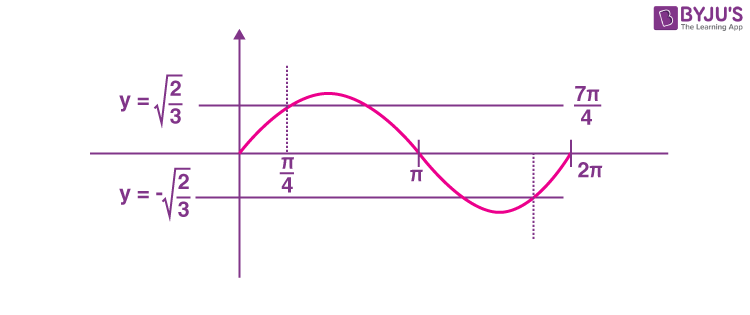$$\begin{array}{l}\sin~x=\pm\sqrt{\frac{2}{3}}\end{array}$$
has 4 solutions in
$$\begin{array}{l}\left ( \frac{\pi}{4},\frac{7\pi}{4} \right )\end{array}$$

6. For a natural number n, let αn = 19n – 12n. Then, the value of

$$\begin{array}{l}\frac{31\alpha_9-\alpha_{10}}{57\alpha_8}\end{array}$$
is ______________.

Sol. αn = 19n – 12n

Let equation of roots 12 & 19 i.e.

$$\begin{array}{l}x^2-31x+228=0\end{array}$$

$$\begin{array}{l}\left ( 31-x \right )=\frac{228}{x}\end{array}$$
(where x can be 19 or 12)

$$\begin{array}{l}\frac{31\alpha_9-\alpha_{10}}{57\alpha_8}=\frac{31\left ( 19^9-12^9 \right )-\left ( 19^{10}-12^{10} \right )}{57\left ( 19^8-12^8 \right )}\end{array}$$
$$\begin{array}{l}=\frac{19^9\left ( 31-19 \right )-12^9\left ( 31-12 \right )}{57\left ( 19^8-12^8 \right )}\end{array}$$
$$\begin{array}{l}=\frac{228\left ( 19^8-12^8 \right )}{57\left ( 19^8-12^8 \right )}=4\end{array}$$
.

7. Let f :RR be a function defined by

$$\begin{array}{l}f\left ( x \right )=\left ( 2\left ( 1-\frac{x^{25}}{2} \right )\left ( 2+x^{25} \right ) \right )^\frac{1}{50}\end{array}$$
. If the function g(x) = f (f (f (x))) + f (f (x)), then the greatest integer less than or equal to g(1) is ___________.

Sol.

$$\begin{array}{l}f\left ( x \right )=\left ( 2\left ( \frac{2-x^{25}}{2} \right )\left ( 2+x^{25} \right ) \right )^\frac{1}{50}\end{array}$$
$$\begin{array}{l}=\left ( 4-x^{50} \right )^\frac{1}{50}\end{array}$$
$$\begin{array}{l}f\left ( f\left ( x \right ) \right )=\left ( 4-\left ( \left ( 4-x^{50} \right )^\frac{1}{50} \right )^{50} \right )^\frac{1}{50}=x\end{array}$$

As f(f(x)) = x we have

g(x) = f(f(f(x))) + f(f(x)) = f(x) + x

g(x) = (4 – x50)1/50 + x

g(1) = 31/50 + 1

⇒ [g(1)] = 2

8. Let the lines

$$\begin{array}{l}L_1:\vec{r}=\lambda\left ( \hat{i}+2\hat{j}+3\hat{k} \right ),\lambda\epsilon R\end{array}$$
$$\begin{array}{l}L_2:\vec{r}=\left ( \hat{i}+3\hat{j}+\hat{k} \right )+\mu\left ( \hat{i}+\hat{j}+5\hat{k} \right );\mu\epsilon R, \end{array}$$

intersect at the point S. If a plane ax + byz + d = 0 passes through S and is parallel to both the lines L1 and L2, then the value of a + b + d is equal to _______.

Sol. As plane is parallel to both the lines we have d.r’s of normal to the plane as < 7, – 2, –1>

$$\begin{array}{l}\left ( \textup{from}\begin{vmatrix}\hat{i} & \hat{j} & \hat{k} \\1 & 2 & 3 \\1 & 1 & 5 \\\end{vmatrix}=7\hat{i}-\hat{j}\left ( 2 \right )+\hat{k}\left ( -1 \right ) \right )\end{array}$$

Also point of intersection of lines is

$$\begin{array}{l}2\hat{i}+4\hat{j}+6\hat{k}\end{array}$$

∴ Equation of plane is

7(x – 2) –2 (y – 4) – 1 (z – 6) = 0

⇒ 7x – 2yz = 0

a + b + d = 7 – 2 + 0 = 5

9. Let A be a 3 × 3 matrix having entries from the set {–1, 0, 1}. The number of all such matrices A having sum of all the entries equal to 5, is ____________.

Sol. Let matrix

$$\begin{array}{l}\begin{bmatrix}a & b & c \\d & e & f \\g & h & i \\\end{bmatrix}\end{array}$$

We need

a + b + c + d + e + f + g + h + i = 5

Possible cases

Number of ways

5 → 1’s, 4 → zeroes

$$\begin{array}{l}\frac{9!}{5!4!}=126\end{array}$$

6 → 1’s, 2 → zeroes, 1 →–1

$$\begin{array}{l}\frac{9!}{6!2!}=252\end{array}$$

7 → 1’s, 2 →–1’s

$$\begin{array}{l}\frac{9!}{7!2!}=36\end{array}$$

Total ways = 126 + 252 + 36 = 414

10. The greatest integer less than or equal to the sum of first 100 terms of the sequence

$$\begin{array}{l}\frac{1}{3},\frac{5}{9},\frac{19}{27},\frac{65}{81},\dots\end{array}$$
is equal to _______________.

Sol.

$$\begin{array}{l}S=\frac{1}{3}+\frac{5}{9}+\frac{19}{27}+\frac{65}{81}+\dots\end{array}$$
$$\begin{array}{l}=\displaystyle\sum\limits_{r=1}^{100}\left ( \frac{3^r-2^r}{3^r} \right )\end{array}$$
$$\begin{array}{l}=100-\frac{2}{3}\frac{\left ( 1-\left ( \frac{2}{3} \right )^{100} \right )}{1/3}\end{array}$$
$$\begin{array}{l}=98+2\left ( \frac{2}{3} \right )^{100}\end{array}$$

[S] = 98

❑ ❑ ❑